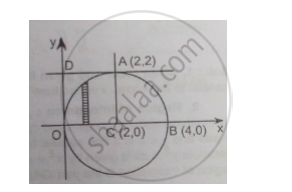Advertisement Remove all ads

# Change the Order of Integration and Evaluate ∫ 2 0 ∫ 2 + √ 4 − Y 2 2 − √ 4 − Y 2 D X D Y - Applied Mathematics 2

Change the order of integration and evaluate int_0^2 int_(2-sqrt(4-y^2))^(2+sqrt(4-y^2)) dxdy

Advertisement Remove all ads

#### Solution

1) Given order and given limits: Given order is is: first w.r.t. x and then w.r.t y i.e., a strip parallel to the x-axis varies from x=2-sqrt(4-y^2) to x=2- sqrt(4-y^2).y  varies from y = 0 to y = 2.

2) Region of integration: x=2-sqrt(4-y^2) is the arc and x =2+sqrt(4-y^2) is the arc of the circle (x-2)^2+y^2=4 4with centre at (2, 0) and radius = 2 above the x-axis. y = 0 is the x-axis and y = 2 is the line parallel to the x-axis through A (2, 2). The region of integration is the semi-circle OAB above the x-axis. The points of intersection of the circle and the x-axis are O (0, 0) and B (4, 0).

3) Change of order of integration: To change the order, consider a strip parallel to the y-axis in the region of integration. On this strip y varies from y = 0 to y=sqrt4-(x-2)^2 and then strip moves from x = 0 to x = 4I= int_0^4 int_0^sqrt(4-(x-2)^2) dydx

I=int_0^4 [y]_0^sqrt(4-(x-2)^2 dx)

I=int_0^4 sqrt(4-(x-2)^2) dx

I=[x-2/2 sqrt(4-(x-2)^2)+2sin ^ -1  (x-2)/2]_0^4

I= (2.pi/2)-(-2. pi/2)

∴ I=2pi

Concept: Application of Double Integrals to Compute Volume
Is there an error in this question or solution?
Advertisement Remove all ads

#### APPEARS IN

Advertisement Remove all ads
Advertisement Remove all ads
Share
Notifications

View all notifications

Forgot password?